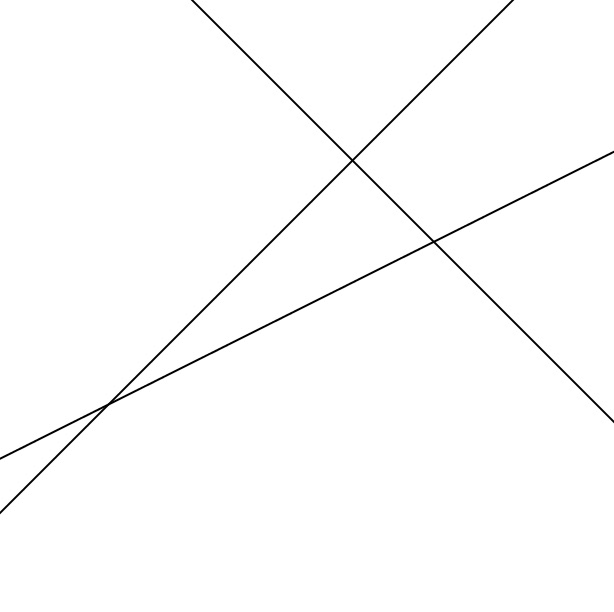What is the area, (in square units) of the triangle formed by the three lines whose equations are: y x = 6x 2y = and  x + y = 6?

This problem is adapted from the NRICH task Graphical Triangle with permission of the University of Cambridge. All rights reserved.